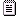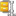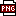#### Plotting Latitude, Longitude, and Elevation

Posted By Sean Dorsett 5 Years Ago

## Plotting Latitude, Longitude, and Elevation

 Author Message Sean DorsettPosted 5 Years AgoForum NewbieGroup: Forum Members Last Active: 3 Years Ago Posts: 9, Visits: 55 I am having some confusion with plotting latitude, longitude, and elevation via the NMeshSurfaceSeries. I see that we have to provide indexX, and indexZ values. I am not entirely clear on why. From what I gather, the values are for an array of sorts? The data I am using is  a comma delimited file containing latitude, longitude, and elevation. How would the data be translated in the method provided below? Thanks in advance for your help. Latitude, Longitude, Depth3357.424,-8648.6006,13357.4242,-8648.6004,13357.4245,-8648.6001,1.13357.4242,-8648.6004,1.23357.4248,-8648.6,1.33357.4248,-8648.6,0.63357.4254,-8648.5997,0.63357.4254,-8648.5997,0.63357.4254,-8648.5997,0.63357.4254,-8648.5997,0.63357.4254,-8648.5997,0.63357.4265,-8648.5976,0.63357.4266,-8648.5971,0.73357.4268,-8648.5965,0.73357.4268,-8648.5965,0.73357.4268,-8648.5965,0.73357.427,-8648.5955,0.73357.427,-8648.5955,0.73357.4273,-8648.5943,0.83357.4273,-8648.5943,0.8         private void FillData(NMeshSurfaceSeries surface)        {            //double x, y, z;            //int nCountX = surface.Data.GridSizeX;            //int nCountZ = surface.Data.GridSizeZ;             //for (int j = 0; j < nCountZ; j++)            //{            //    for (int i = 0; i < nCountX; i++)            //    {            //        x = 2 + i + Math.Sin(j / 4.0) * 2;            //        z = 1 + j + Math.Cos(i / 4.0);             //        y = Math.Sin(i / 3.0) * Math.Sin(j / 3.0);             //        if (y < 0)            //        {            //            y = Math.Abs(y / 2.0);            //        }             //        System.Diagnostics.Debug.WriteLine("{0},{1},{2},{3}", j, i, y, x, z);            //        surface.Data.SetValue(j, i, y, x, z);            //    }            //}             surface.Data.SetValue(j, i, y, x, z);         } Sean Dorsett Posted 5 Years AgoForum NewbieGroup: Forum Members Last Active: 3 Years Ago Posts: 9, Visits: 55 So I was able to determine that the data values were in degrees and minutes. So the first point 3357.424,-8648.6006,1 translate into latitude = 33.9570666, longitude = -86.81001, elevation = 1m. I was able to convert the latitude/longitude to cartesian coordinates for plotting a surface chart. Unfortunately, the data does not plot a smooth mesh surface series. I have attached my surface plot and the data used.AttachmentsTestData.txt (433 views, 29.00 KB) Nevron Support Posted 5 Years AgoSupreme BeingGroup: Forum Members Last Active: Yesterday @ 1:05 AM Posts: 2,965, Visits: 3,372 Hi Sean,Do you have an image of how this data should look like? If you send the coordinate transformation you work with we can also check what is the best way to present this data - it looks like that the data does not have an order so you should probably use the triangulated surface series (which accepts a set of 3D points and creates a surface by connecting them). Best Regards,Nevron Support Team Sean Dorsett Posted 5 Years AgoForum NewbieGroup: Forum Members Last Active: 3 Years Ago Posts: 9, Visits: 55 Hello and thanks for the response. I figured out that I need to use a triangulated surface. I am including the code I wrote to generate the contour plot. At this point, I have two questions for you.1) How can I display a horizontal plane at the 0th elevation. If you look at my code, I attempted to display a plane by simply obtaining the max and min X and Z values and setting their depth to zero. The results were not favorable. In one of the images provided by way of another plotting application, you can see the horizontal plane.2) Is there a limitation on the number of data points to plot? When attempting to plot the data in the attached file LargeNumberOfData.csv, I get a Stack Overflow error. "An unhandled exception of type 'System.StackOverflowException' occurred in Nevron.Presentation.dll"Lastly, I should point out that in my could I have added "Convert.ToDouble(point) <= 5)" to deal with the data outlier issue. I suspect that I will have to add some logic to clean my data of outliers before plotting..using Nevron.Chart;using Nevron.Chart.Windows;using Nevron.GraphicsCore;using System;using System.Collections.Generic;using System.Drawing;using System.IO;using System.Windows.Forms;namespace WindowsFormsApplication4{  public partial class Form1 : Form  {   public Form1()   {    InitializeComponent();    nChartControl1.Settings.ShapeRenderingMode = ShapeRenderingMode.None;    nChartControl1.Controller.Tools.Add(new NSelectorTool());    nChartControl1.Controller.Tools.Add(new NTrackballTool());    // set a chart title    NLabel title = nChartControl1.Labels.AddHeader("Mesh Surface Chart");    title.TextStyle.FontStyle = new NFontStyle("Times New Roman", 18, FontStyle.Italic);    title.TextStyle.FillStyle = new NColorFillStyle(Color.AliceBlue);    // setup chart    NChart chart = nChartControl1.Charts;    chart.Enable3D = true;    chart.Width = 70.0f;    chart.Depth = 70.0f;    chart.Height = 30.0f;    chart.Projection.SetPredefinedProjection(PredefinedProjection.PerspectiveTilted);    // setup axes    NLinearScaleConfigurator linearScale = new NLinearScaleConfigurator();    linearScale.MajorGridStyle.SetShowAtWall(ChartWallType.Floor, true);    linearScale.MajorGridStyle.SetShowAtWall(ChartWallType.Back, true);    linearScale.RoundToTickMax = false;    linearScale.RoundToTickMin = false;    chart.Axis(StandardAxis.PrimaryX).ScaleConfigurator = linearScale;    linearScale = new NLinearScaleConfigurator();    linearScale.MajorGridStyle.SetShowAtWall(ChartWallType.Floor, true);    linearScale.MajorGridStyle.SetShowAtWall(ChartWallType.Left, true);    linearScale.RoundToTickMax = false;    linearScale.RoundToTickMin = false;    chart.Axis(StandardAxis.Depth).ScaleConfigurator = linearScale;    // setup surface series    NTriangulatedSurfaceSeries surface = new NTriangulatedSurfaceSeries();    surface.Name = "Surface";    surface.Legend.Mode = SeriesLegendMode.SeriesLogic;    surface.FillMode = SurfaceFillMode.ZoneTexture;    surface.ShadingMode = ShadingMode.Smooth;    surface.FrameMode = SurfaceFrameMode.None;    surface.FrameColorMode = SurfaceFrameColorMode.Zone;    surface.DrawFlat = false;    surface.PositionValue = 0.5;    //surface.Data.SetGridSize(20, 20);    surface.SyncPaletteWithAxisScale = false;    surface.PaletteSteps = 8;    surface.ValueFormatter.FormatSpecifier = "0.00";    surface.FillStyle = new NColorFillStyle(Color.YellowGreen);    chart.Series.Add(surface);    List geospatialPoints = GetSurveyData();    for (int i = 0; i < geospatialPoints.Count; i++)    {      surface.Values.Add(geospatialPoints[i].y);      surface.XValues.Add(geospatialPoints[i].x);      surface.ZValues.Add(geospatialPoints[i].z);        }    nChartControl1.Refresh();   }      private List GetSurveyData()   {        List geospatialPoints = new List();    try    {       // Open the text file using a stream reader.      using (StreamReader sr = new StreamReader("TestData.csv"))      {       string headerLine = sr.ReadLine();              string line;       double minX = 0;       double maxX = 0;       double minZ = 0;       double maxZ = 0;       // Read the stream to a string.       while ((line = sr.ReadLine()) != null)       {        if (line.Contains(","))        {          string[] point = line.Split(',');          if (IsValidGeospatialPoint(point) && Convert.ToDouble(point) <= 5)          {           double latitude = ConvertDegreeAngleToDouble(point);           double longitude = ConvertDegreeAngleToDouble(point);           double elevation = Convert.ToDouble(point);           double cosLat = Math.Cos(latitude * Math.PI / 180.0);           double sinLat = Math.Sin(latitude * Math.PI / 180.0);           double cosLon = Math.Cos(longitude * Math.PI / 180.0);           double sinLon = Math.Sin(longitude * Math.PI / 180.0);           double rad = 6378137.0;           double f = 1.0 / 298.257224;           double C = 1.0 / Math.Sqrt(cosLat * cosLat + (1 - f) * (1 - f) * sinLat * sinLat);           double S = (1.0 - f) * (1.0 - f) * C;           double h = 0;           double cartesianX = ((rad * C + h) * cosLat * cosLon);           double cartesianY = -elevation;           double cartesianZ = ((rad * S + h) * sinLat);           if (geospatialPoints.Find(geoPoint => (geoPoint.x == cartesianX && geoPoint.y == cartesianY && geoPoint.z == cartesianZ)) == null)           {            System.Diagnostics.Debug.WriteLine("{0}, {1}, {2}", cartesianX, cartesianZ, cartesianY);            geospatialPoints.Add(new GeospatialPoint()            {              x = cartesianX,              y = cartesianY,              z = cartesianZ            });           }          }        }       }              //minX = (from p in geospatialPoints select p.x).Min();       //minZ = (from p in geospatialPoints select p.z).Min();       //maxX = (from p in geospatialPoints select p.x).Max();       //maxZ = (from p in geospatialPoints select p.z).Max();       //geospatialPoints.Add(new GeospatialPoint() { x = minX, z = minZ, y = 0 });       //geospatialPoints.Add(new GeospatialPoint() { x = maxX, z = minZ, y = 0 });       //geospatialPoints.Add(new GeospatialPoint() { x = maxX, z = maxZ, y = 0 });       //geospatialPoints.Add(new GeospatialPoint() { x = minX, z = maxZ, y = 0 });      }    }    catch (Exception e)    {      Console.WriteLine("The file could not be read:");      Console.WriteLine(e.Message);    }    return geospatialPoints;   }   private double ConvertDegreeAngleToDouble(string degreesMinutes)   {    // Decimal degrees =     // whole number of degrees,     // plus minutes divided by 60,     // plus seconds divided by 3600    // 3357.4196    // degrees = 33    // minutes = 57.4196    string[] degreesMinutesDivided = degreesMinutes.Split('.');    string degrees = degreesMinutesDivided.Substring(0, degreesMinutesDivided.Length - 2);    string minutes = degreesMinutesDivided.Substring(degrees.Length ) + "." + degreesMinutesDivided;    string seconds = "0";    return Convert.ToDouble(degrees) + (Convert.ToDouble(minutes) / 60) + (Convert.ToDouble(seconds) / 3600);   }   private bool IsValidGeospatialPoint(string[] point)   {    if (point.Length >= 3)    {      double latitude;      double longitude;      double elevation;      if (Double.TryParse(point, out latitude) && Double.TryParse(point, out longitude) && Double.TryParse(point, out elevation))      {       return true;      }      return false;    }    return false;   }  }} AttachmentsTriangulate.zip (376 views, 96.00 KB) Sean Dorsett Posted 5 Years AgoForum NewbieGroup: Forum Members Last Active: 3 Years Ago Posts: 9, Visits: 55 I am attaching a copy of what the plot should look like. I am also including images of what I rendered via the Nevron interface. The data for the plot is from the TestData.csv file. Attachmentsimg1.png (780 views, 46.00 KB)img2.png (605 views, 255.00 KB)img3.png (602 views, 161.00 KB) Nevron Support Posted 5 Years AgoSupreme BeingGroup: Forum Members Last Active: Yesterday @ 1:05 AM Posts: 2,965, Visits: 3,372 Hi Sean,We think that the transformation produces incorrect points that's why you get such results. Please find attached a sample app that shows how to plot the same surface using grid and triangulated surface series. The reference image you attached is most likely displayed with grid surface equivalent as the x/z coordinates look equally spaced. Regarding your question:1) The sample app shows that there is nothing special about the flat surface - simply the y coordinates of adjacent points are equal.2) There is no internal limitation to the number of data points in the surface - some of our users use the control with millions of data points. The exception is very strange given the fact that VS does not show the stack trace and only appears when running the app in debug mode so it may be related to a .NET bug and not the control.Hope this helps - let us know if you meet any problems or have any questions. Best Regards,Nevron Support Team AttachmentsSurfaceTest.zip (364 views, 11.00 KB) Sean Dorsett Posted 5 Years AgoForum NewbieGroup: Forum Members Last Active: 3 Years Ago Posts: 9, Visits: 55 Thanks for the sample application. I was hoping for some sort of example that used the data I provided.Your answer for issue #2 is not going to fly for me. The error clearly states that there is a problem in the Nevron.Presentation.dll. Am I missing something here? I cannot have my client purchase your software if I can expect a stack overflow exception when plotting data. The file I provided has no more than 2400 data points. I will need a more concrete answer here.System.StackOverflowException was unhandledMessage: An unhandled exception of type 'System.StackOverflowException' occurred in Nevron.Presentation.dll Nevron Support Posted 5 Years AgoSupreme BeingGroup: Forum Members Last Active: Yesterday @ 1:05 AM Posts: 2,965, Visits: 3,372 Hi Sean,We just made some more tests and the problem goes away if you use precise floating point arithmetic:surface.UsePreciseGeometry = true;this this is most likely caused by triangulation which by default relies on standard floating point arithmetic which is faster but can lead to loss of precision in certain calculations.Regarding the surface - it is not clear what the transformation of the points should be - we're certain that the chart displays them correctly it's just that they have weird coordinates. Best Regards,Nevron Support Team